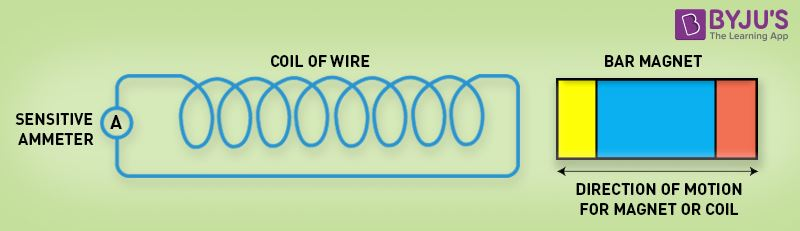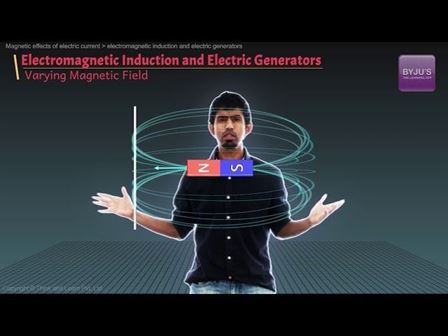# Faraday’s Laws of Electromagnetic Induction

Faraday’s law of electromagnetic induction, also known as Faraday’s law is the basic law of electromagnetism which helps us predict how a magnetic field would interact with an electric circuit to produce an electromotive force (EMF). This phenomenon is known as electromagnetic induction.

The law was proposed in the year 1831 by an experimental physicist and chemist named Michael Faraday. So you can see where the name of the law comes from. That being said, the Faraday’s law or the law of electromagnetic induction is basically the results or the observations of the experiments that Faraday conducted. He performed three main experiments to discover the phenomenon of electromagnetic induction.

## Faraday’s Laws of Electromagnetic Induction

Faraday’s Laws of Electromagnetic Induction consists of two laws. The first law describes the induction of emf in a conductor and the second law quantifies the emf produced in the conductor. In the next few sections, let us learn these laws in detail.

### Faraday’s First Law of Electromagnetic Induction

The discovery and understanding of electromagnetic induction are based on a long series of experiments carried out by Faraday and Henry. From the experimental observations, Faraday arrived at a conclusion that an emf is induced in the coil when the magnetic flux across the coil changes with time. With this in mind, Faraday formulated his first law of electromagnetic induction as,

Whenever a conductor is placed in a varying magnetic field, an electromotive force is induced. If the conductor circuit is closed, a current is induced which is called induced current.

### Changing the Magnetic Field Intensity in a Closed LoopMentioned here are a few ways to change the magnetic field intensity in a closed loop:

• By rotating the coil relative to the magnet.
• By moving the coil into or out of the magnetic field.
• By changing the area of a coil placed in the magnetic field.
• By moving a magnet towards or away from the coil.

## Faraday’s Second Law of Electromagnetic Induction

Faraday’s second law of electromagnetic induction states that

The induced emf in a coil is equal to the rate of change of flux linkage.

The flux is the product of the number of turns in the coil and the flux associated with the coil. The formula of Faraday’s law is given below:

<tbody

 $\varepsilon =-N\frac{\Delta \phi }{\Delta t}$

Where,

• ε is the electromotive force
• Φ is the magnetic flux
• N is the number of turns

The negative sign indicates that the direction of the induced emf and change in direction of magnetic fields have opposite signs.

Additionally, there is another key law known as Lenz’s law that describes electromagnetic induction as well.

Consider a magnet approaching towards a coil. Consider two time instances T1 and T2.

Flux linkage with the coil at the time T1 is given by

T1 = NΦ1

Flux linkage with the coil at the time T2 is given by

T1 = NΦ2

Change in the flux linkage is given by

N(Φ2 – Φ1)

Let us consider this change in flux linkage as

Φ = Φ2 – Φ1

Hence, the change in flux linkage is given by

The rate of change of flux linkage is given by

NΦ/t

Taking the derivative of the above equation, we get

N dΦ/dt

According to Faraday’s second law of electromagnetic induction, we know that the induced emf in a coil is equal to the rate of change of flux linkage. Therefore,

$E=N\frac{d\phi }{dt}$

Considering Lenz’s law,

$E=-N\frac{d\phi }{dt}$

From the above equation, we can conclude the following

• Increase in the number of turns in the coil increases the induced emf
• Increasing the magnetic field strength increases the induced emf
• Increasing the speed of the relative motion between the coil and the magnet, results in the increased emf

Learn more about Faraday’s Law of induction and the relationship between the electric circuit and magnetic field by watching this engaging video from BYJU’S.## Faraday’s Experiment: Relationship Between Induced EMF and Flux

• In the first experiment, he proved that when the strength of the magnetic field is varied, only then current is induced. An ammeter was connected to a loop of wire; the ammeter deflected when a magnet was moved towards the wire.
• In the second experiment, he proved that passing a current through an iron rod would make it electromagnetic. He observed that when a relative motion exists between the magnet and the coil, an electromotive force will be induced. When the magnet was rotated about its axis, no electromotive force was observed but when the magnet was rotated about its own axis then the induced electromotive force was produced. Thus, there was no deflection in the ammeter when the magnet was held stationary.

• While conducting the third experiment, he recorded that galvanometer did not show any deflection and no induced current was produced in the coil when the coil was moved in a stationary magnetic field. The ammeter deflected in the opposite direction when the magnet was moved away from the loop.

Summarising the above points, in a table we have mapped out the relationship between the position of magnet and the deflection in Galvanometer.

 Position of Magnet Deflection in Galvanometer Magnet at Rest No deflection in the galvanometer The magnet moves towards the coil Deflection in the galvanometer in one direction Magnet is held stationary at the same position( near the coil) No deflection galvanometer The magnet moves away from the coil Deflection in galvanometer but in the opposite direction The magnet held stationary at the same position(away from the coil) No deflection in the galvanometer

### Conclusion:

After conducting all the experiments, Faraday finally concluded that if relative motion existed between a conductor and a magnetic field, the flux linkage with a coil changed and this change in flux produced a voltage across a coil.

Faraday law basically states, “when the magnetic flux or the magnetic field changes with time, the electromotive force is produced”. Additionally, Michael Faraday also formulated two laws on the basis of the above experiments.

Following are the fields where Faraday’s law find applications:

1. Electrical equipment like transformers work on the basis of Faraday’s law.
2. Induction cooker works on the basis of mutual induction which is the principle of Faraday’s law.
3. By inducing an electromotive force into an electromagnetic flowmeter, the velocity of the fluids is recorded.
4. Electric guitar and electric violin are the musical instruments that find an application of Faraday’s law.
5. Maxwell’s equation is based on the converse of Faraday’s laws which states that change in the magnetic field brings a change in the electric field.

### What is Faraday’s law of mutual induction?

When an emf is produced in a coil because of the change in current in a coupled coil, the effect is called mutual inductance.

### What is meant by EMF?

Electromotive force or emf is a measurement of the energy that causes current to flow through a circuit.

### Why is Faraday’s law important?

Faraday’s law looks at how changing magnetic fields can cause current to flow in wires.

### What are the methods to change the magnetic field in the Faraday’s first law?

According to Faraday’s first law, any minute change in the magnetic field of the coil results in emf which is induced in the coil. Following are the ways in which the magnetic field can be changed:

• The movement of the bar magnet towards or away from the coil.
• The movement of the coil into or out of the magnetic field.
• Changing the area where the coil is placed results in a change in the magnetic field.
• The rotation of the coil relative to the magnet brings the change in the magnetic field.

### How can we say that the magnetic flux is zero when the magnetic field is not zero?

The relation between the magnetic flux and the magnetic field is given as:
Φ = BAcosθ
When cosθ is zero, then the magnetic flux will be zero irrespective of the presence of the magnetic field. Therefore, when the magnetic field is parallel to the surface, the magnetic flux will be equal to zero.

The negative sign in Faraday’s law of electromagnetic induction formula indicates the direction of the induced field. The direction of the induced field can be remembered by understanding the Lenz’s law. According to Lenz’s law, current flow is the result of emf which is produced in the magnetic field which opposes the change in the flux that produces it.

### How to increase emf induced in a coil?

• By increasing the number of turns in the coil that is N, induced emf also increases.
• By increasing the magnetic field strength B which surrounds the coil, increases the flux which thereby increases the emf induced in the coil.
• The relative motion between the coil and the magnet should have an increase in the speed which results in cutting off the lines of flux at a faster rate and so the induced emf would be more.